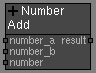#### Pixaflux

Left Toolbar >Math Nodes >Number Add

The Number Add node allows you to execute an addition operation on multiple Number values.

## Procedure

### To execute an addition operation on multiple Node Values in the node graph:

1. On the left toolbar choose Math Nodes> Number Add2. Drag the Number Add icon into the Node Graph.3. Connect the Number Node to the inputs to be added, or set its value in the attributes panel manually.

4. Connect the Output: result.

## Inputs

number A NUMBER type of value. This input grows automatically when a value is connected.

## Outputs

number The number value.

## Attributes Panelnumber_a The first term in the addition operation. This attribute is exposed as input by default.

number_b The second term in the addition operation. This attribute is exposed as input by default.

Math Nodes

Main Page